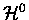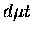Next: Variational approaches Up: Adiabatic approaches Previous: Multi-level calculations

The difficulties associated with the Adiabatic Representation method have been discussed by many authors (for example, see Ref. ). Ponomarev summarizes its disadvantages in treating boundary conditions [103,104]:

1.
Incorrect dissociation limit of the system, when it decays intoand.
2.
Incorrect value of momentum in the reaction channels.
3.
Infinite and long-range behavior of the non-diagonal potential Uij(R) as.

Cohen and his co-workers at Los Alamos used what they call the Improved Adiabatic'' approach  to address some of the above limitations. In the standard Adiabatic Representation method, the Hamiltonian was separated into two parts (Eq. 2.5); the zero order term describing the two centre Coulomb problem (TC), and the perturbative term for the relative motions of the nuclei as well as nuclear-muonic motion coupling (). In the asymmetric nuclear system (e.g., Ma > Mb), since infinite mass for both the nuclei is assumed inTC, the zeroth order solution cannot distinguish the channelfrom, into the former of which the ground muonic state () should dissociate in the limit(recall theis more deeply bound than thedue to the reduced mass difference). The Improved Adiabatic approach instead partitions the Hamiltonian into==(31)

where the mass polarization (i.e. nuclear-muonic motion coupling) termand the internuclear orientation term, which were part of the perturbationin the Adiabatic Representation, are now included in the zero order Hamiltonian. The termacting in the nuclear centre of mass frame (as opposed to the geometric centre of nuclei frame) breaks the symmetry, and causes the zero order muon wave function to move to the heavier nuclei as. Therefore, the Improved Adiabatic method is expected to give more accurate results for the asymmetric nuclear case, compared to the standard Adiabatic Representation approximation, for the same number of basis functions N, hence achieving faster convergence. Of course, in the limit, both approaches should give the same results. The two level approximation of the Improved Adiabatic method has been applied by the Los Alamos group to the bound state calculations of HD+  and, as well as in the scattering calculations [19,20,21]. The latter cover all the possible combinations of muonic hydrogen isotope scattering, including the only calculation to date of the hyperfine transitions in asymmetric collisions.

Despite the criticisms of the Adiabatic Representation, it should be noted that the effective two level approximation used by Bubak and Faifman (p.) also deals with the dissociation problem within the framework of the Adiabatic Representation, and appears to give a reasonable accuracy for only N=2.Next: Variational approaches Up: Adiabatic approaches Previous: Multi-level calculations